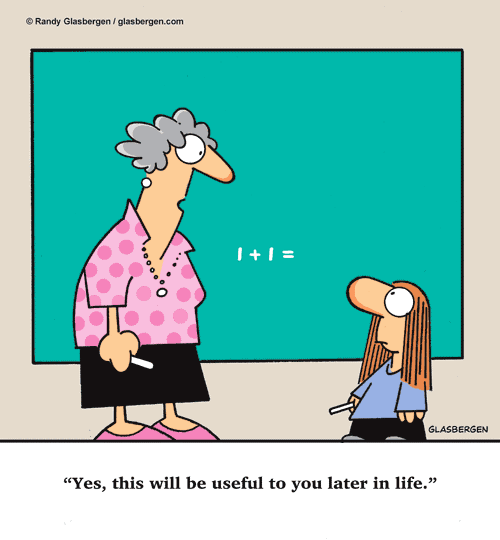•Mrs. GuymonTERRI GUYMON – 7TH & 8TH GRADE MATH

• Bachelor of Science in Elementary Education from SIUE
• Has been teaching at Evangelical School for 12 years
• Likes to read and spend time with family and friends

Hello! I’m  Terri Guymon and this is my eleventh year teaching middle school mathematics here at Evangelical School. We are off to a great start this year. We started our year with  Random Rocks of Math. I have hidden 25 rocks in the 7th and 8th grade rooms for students to find and bring to me for the math problem that goes with that rock. I haven’t yet decided what the prize will be for the most correct answers. I’m sure the students will have some good ideas.

Thank you to all who have donated drinks for our Math Mania Mondays. The students are enjoying Math Mania.Seventh grade students will be in one of two math classes.

In Pre-Algebra (Prentice Hall), students will begin making a more formal transition from concrete mathematics into algebra and geometry. They will study topics that include: algebraic expressions and integers, solving one-step equations and inequalities, decimals, factors, fractions, exponents, operations with fractions, ratios, proportions, percents, solving multiple step equations and inequalities, linear functions and graphing, spatial thinking, area, volume, and right triangles in algebra.

In Mathematics Course 2 (Prentice Hall), we will study the following topics in more depth: decimals, integers, exponents, factors, fractions, ratios, rates, proportions, percents, measurement, geometry, and beginning algebra concepts.